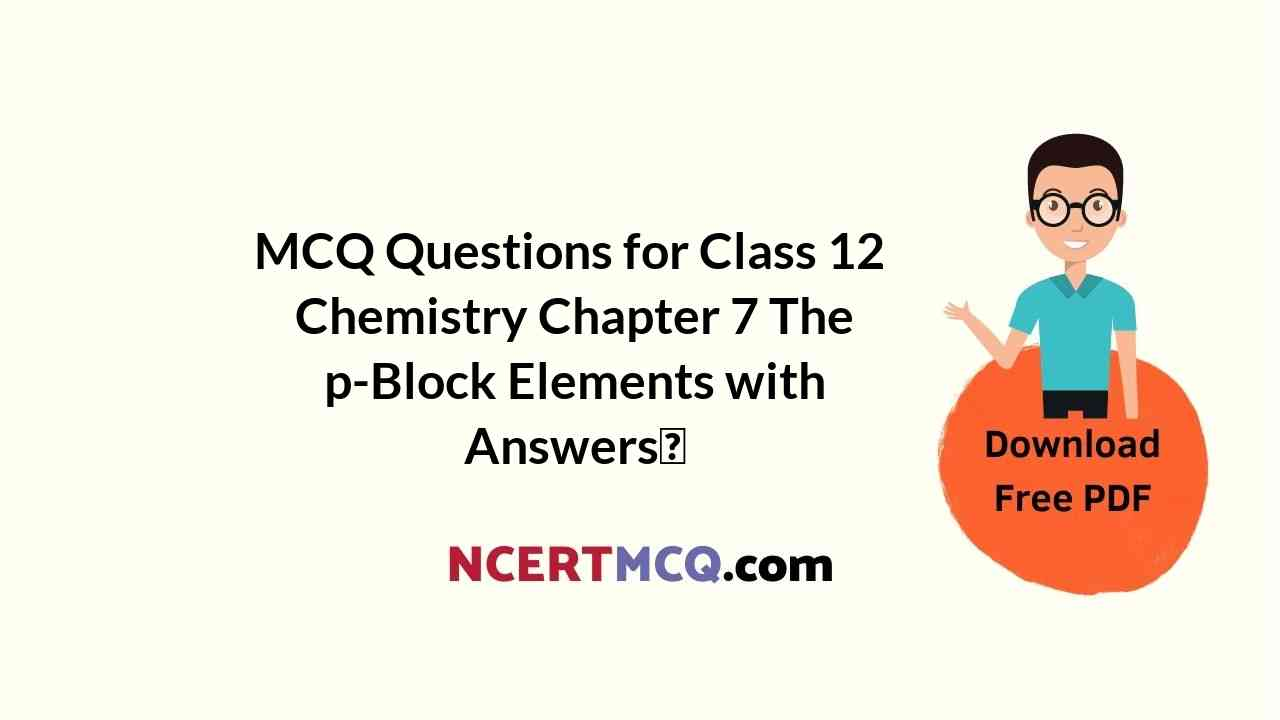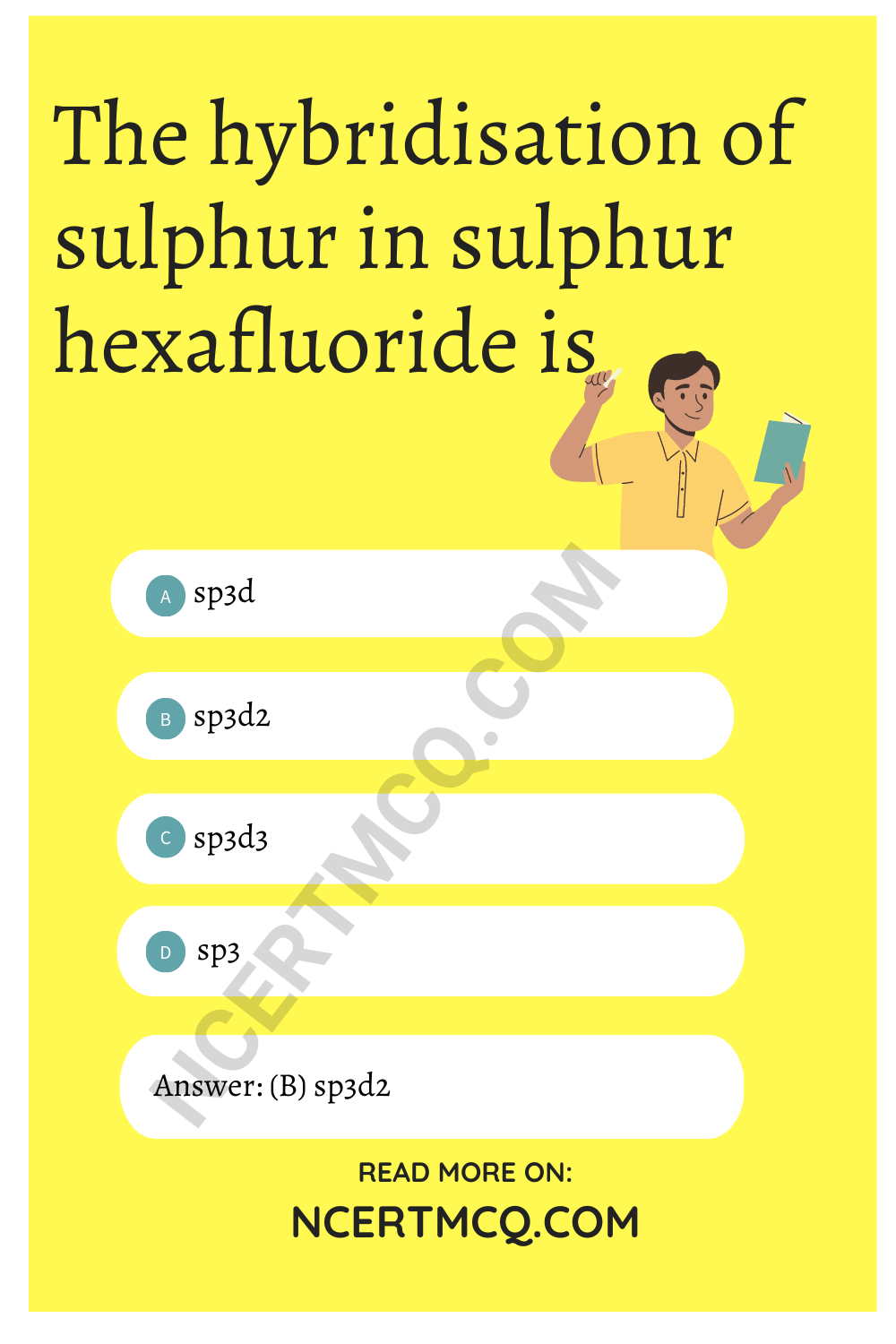Check the below NCERT MCQ Questions for Class 12 Chemistry Chapter 7 The p-Block Elements with Answers Pdf free download. MCQ Questions for Class 12 Chemistry with Answers were prepared based on the latest exam pattern. We have provided The p-Block Elements Class 12 Chemistry MCQs Questions with Answers to help students understand the concept very well.

## The p-Block Elements Class 12 MCQs Questions with Answers

P Block Elements Class 12 MCQ Question 1.
Boron shows diagonal relation with
(a) Al
(b) C
(c) Si
(d) Sn

MCQ Of P Block Elements Class 12 Question 2.
Catenation property is maximum in
(a) phosphorus
(b) carbon
(c) sulphur
(d) zinc

MCQ On P Block Elements Class 12 Question 3.
Which one of the following is an electrophilic reagent?
(a) BF3
(b) KH3
(c) H2O
(d) None of these

P Block Elements MCQ Question 4.
Element found from sea water is
(a) magnesium
(b) Sodium
(c) Iodine
(d) None of theseP Block MCQ Class 12 Question 5.
The shape of XeF4 is
(a) tetrahedral
(b) square planar
(c) pyramidal
(d) linear

P Block Class 12 MCQ Question 6.
Which one of the following is the strongest lewis acid?
(a) BF3
(b) BCl3
(c) BBr3
(d) Bl3

Class 12 P Block MCQ Question 7.
Which one of the following does not form hydrogen bonding?
(a) NH3
(b) H2O
(c) HCl
(d) HF

P Block Elements MCQ Class 12 Question 8.
Main source of helium is
(a) Air
(c) Monazite
(d) Water

Class 12 Chemistry P Block MCQ Question 9.
Which one of the following dement is liquid at normal temperature?
(a) zinc
(b) mercury
(c) bromine
(d) water

Class 12 Chemistry Chapter 7 MCQ Question 10.
Which one of the following is least basic?
(a) NCl3
(b) NBr3
(c) NI3
(d) NF3

P Block Elements Class 12 MCQs Question 11.
H2SO4 is a/an
(a) acid
(b) base
(c) alkali
(d) salt

P Block Elements MCQs Question 12.
Modern periodic table is given by
(a) Debonair
(b) Mendeleef
(c) Mendel
(d) None of these

Class 12 P Block Elements MCQ Question 13.
Which one of the following elements is found in free state in nature?
(a) Sodium
(b) Iron
(c) Zinc
(d) Gold

MCQs On P Block Elements Class 12 Question 14.
Which of the following is the strongest oxidising agent?
(a) F2
(b) Cl2
(c) l2
(d) Br2

P Block Elements Class 12 MCQ Questions Question 15.
Which of the following has maximum ionisation potential?
(a) Al
(b) P
(c) Si
(d) Mg

Question 16.
The number of P-O-P bonds in cyclic metaphosphoric acid is
(a) 2
(b) 0
(c) 3
(d) 4

Question 17.
Which of the following oxides is an hydride of nitrous acid ?
(a) N2O3
(b) NO2
(c) NO
(d) N2O4

Question 18.
Which oxide of nitrogen is obtained on heating ammonium nitrate at 250°C ?
(a) Nitric oxide
(b) Nitrous oxide
(c) Nitrogen dioxide
(d) Dinitrogen tetraoxide

Question 19.
Atomicity of phosphorus is
(a) 1
(b) 2
(c) 3
(d) 4

Question 20.
The structure of white phosphorus is
(a) square-planar
(b) pyramidal
(c) tetrahedral
(d) trigonal planar

Question 21.
PCl3 on hydrolysis gives
(a) H3PO3
(b) HPO3
(c) H3PO4
(d) POCl3

Question 22.
Arrange the following in decreasing Lewis acid strength -PF3, PCI3, PBr3, Pl3
(a) Pl3 > PBr3 > PCl3 > PF3
(b) PF3 > PCl3 > PBr3 > Pl3
(c) PCl3 > PBr3 > Pl3 > PF3
(d) PBr3 > Pl3 > PF3 > PCl3

Answer: (a) Pl3 > PBr3 > PCl3 > PF3

Question 23.
How many P-O-P bonds appear in cyclic meta-phosphoric acid?
(a) 4
(b) 3
(c) 2
(d) 1

Question 24.
Which of the following is a tetrabasic acid?
(a) Hypophosphorus acid
(b) Metaphosphoric acid
(c) Pyrophosphoric acid
(d) Orthophosphoric acid

Question 25.
Which of the following is not correctly matched?
(a) PCl5-sp3d hybridisation
(b) PCl3-sp3 hybridisation
(c) PCl5 (solid)-[PtCl4] [PtCl3]
(d) H3PO3 -tribasic

Question 26.
Arrange the following hydrides of group 16 elements in order of increasing stability
(a) H2S < H2O < H2Te > H2Se
(b) H2O < H2Te < H2Se < H2S
(c) H2O < H2S < H2Se < H2Te
(d) H2Te < H2Se < H2S < H3O

Answer: (d) H2Te < H2Se < H2S < H3O

Question 27.
The hybridisation of sulphur in sulphur hexafluoride is
(a) sp3d
(b) sp3d2
(c) sp3d3
(d) sp3Question 28.
On heating KCIO, we get
(a) KClO2 + O2
(b) KCl + O2
(c) KCI + O3
(d) KCl + O2 + O3

Question 29.
Which of the following is not correctly matched?
(a) Acidic oxides-P2O5 NO2, CL2O7
(b) Basic oxides-Na2Om, CaO, MgO
(c) Neutral oxides-CO2, CO, BeO
(d) Amphoteric oxides-ZnO, SnO,Al2O3

Answer: (c) Neutral oxides-CO2, CO, BeO

Question 30.
Sulphur molecule is
(a) diatomic
(b) triatamic
(c) tetratomic
(d) Octa-atomic